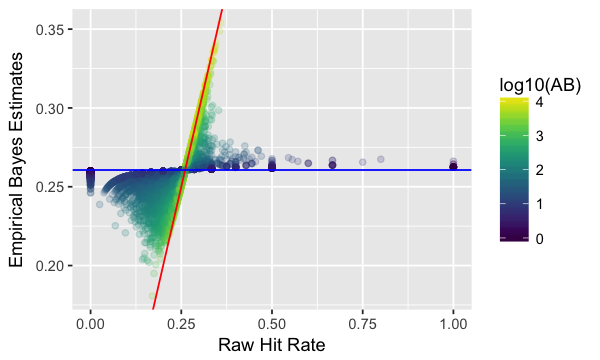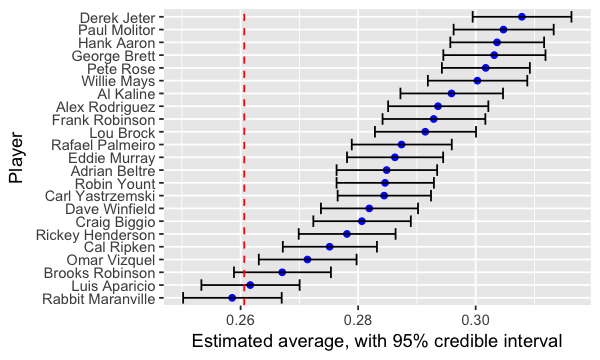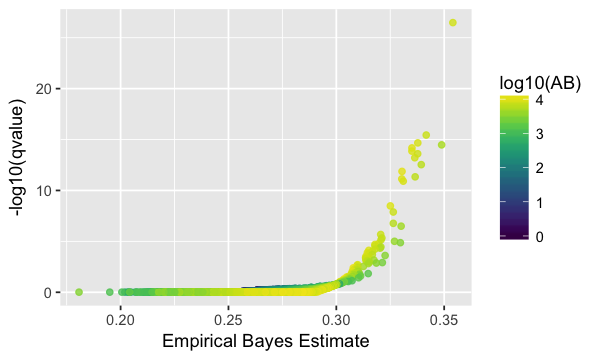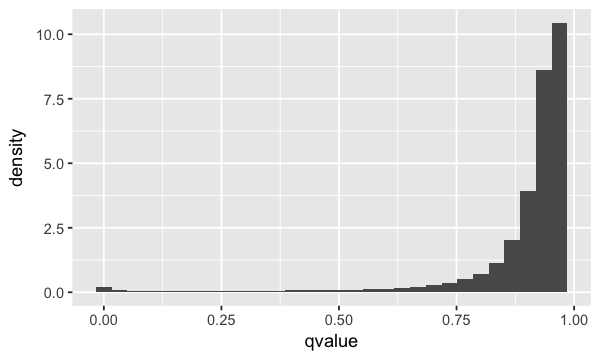# Introduction to Empirical Bayes using Baseball Statistics¶

This post draws extensively on material from the book Introduction to Empirical Bayes by David Robinson. I've found the book to be an excellent introduction to Empirical Bayes, and a great introduction to the Lahman Baseball dataset as well. You can find the book here. I strongly suggest it!

## Empirical Bayes¶

Empirical Bayes refers to a "hacker" approach to a specific kind of Bayesian Analysis. Empirical Bayes can be successfully employed when data can be modelled by a Binomial distribution depending on a parameter $N_i$ and $p_i$, where $p_i$ is the quantity we want to estimate for each data point. Crucially, it may be the case that each point has a specific $p_i$ that is not, in general, equal to any other $p_i$. In that case, we can think of the set $\{p_i\}$ as being drawn from a distribution that has a well-defined mean and variance. If the distribution is not uniform, then the data points should not be considered independent of each other-each point contains information about the prior values of the other points. Therefore, we would like an approach that "draws power" from all the data points to help us perform inference on the most likely value $p_k$ for a data point $k$ for which we may otherwise have limited evidence (for example, if we wanted to know the hit rate for a baseball player who has only played five games, instead of 1,000).

Notice that the above scenario is different from what we often hope for in science. In scientific experiments, we will often set up an experimental design to measure a variable $p$, and the experiment will be replicated several times to generate various measurements of this variable. In this case, each replicate is considered to be a binomial draw using the same coin, $p$, and the variability is attributed to random sampling. These two scenarios are similar, but fundamentally very different. In the data, the way this will play out is in the variance. Tossing many different coins with binomial sampling will result in a greater variance than tossing a single coin with binomial sampling.

Empirical Bayes is the result of placing a Beta prior on a Binomial likelihood and evaluating the result (since the Beta is a conjugate prior for the Binomial, the result is an "updated" Beta distribution). The Beta distribution depends on two hyperparameters, $\alpha$ and $\beta$, and a proper Bayesian calls for priors on these two hyperparameters (and priors on the priors of the priors, etc...), Empirical Bayes will be happy with ignoring any further priors.

# Getting started¶

To start, I will load some default libraries in R, including the Lahman dataset.

In :
library(dplyr)
library(stats4)
library(ggplot2)
library(tidyr)
library(viridis)
library(Lahman)

## set universal plot size:
options(repr.plot.width=5, repr.plot.height=3)

Loading required package: viridisLite


To motivate the problem, let's start with a simulation. Suppose we simulate 1 million baseball players, each of whom have exactly 300 at-bats (chances to hit the ball; I'm not a baseball player). For each player, we will draw a coin from a beta distribution, $p_i \sim \mathrm{Beta}(81, 219, p)$, and then we will simulate their hitting career with a Binomial distribution generated using that coin ($\mathrm{Bin}(N, p_i)$).

In :
# Simulate player statistics. For each player, choose a coin from the beta
# distribution, Beta(81, 219). Then, simulate the number of balls they hit
# out of 300 at-bats, given the coin they were assigned.
num_trial <- 10e6

sims <- data_frame(
true_average = rbeta(num_trial, 81, 219),
hits = rbinom(num_trial, 300, true_average)
)


true_averagehits
0.3137426 81
0.3141298106
0.2944093 97
0.2468624 78
0.2416608 62

Now that we have a dataset, we can ask a range of questions. For example, what are the range of hit-rates ($p_i$'s) of players that hit exactly 100/300 balls?

In :
# look at only players who hit exactly 100/300 balls
hit_100 <- sims %>%
filter(hits == 100)

# Make a histogram of the players who hit 100 balls out of 300 attempts
# Also draw a KDE on top
sims %>%
filter(hits == 100) %>%
ggplot(aes(true_average), color=factor(hits)) +
geom_histogram(aes(y=..density..)) +
geom_density() +
labs(x = 'True average of players wth H hits / 300 total bats',
y = 'Density', color="H")

# Print the mean average for the players who hit 100/300
total <- career_filtered$AB -sum(VGAM::dbetabinom.ab(x, total, alpha, beta, log=TRUE)) } # calculate the maximum likelihood estimate: m <- mle(ll, start=list(alpha=1, beta=10), method="L-BFGS-B", lower=c(0.0001, .1)) # extract the coefficients ab <- coef(m) alpha0 <- ab beta0 <- ab # print coefficients cat(alpha0, beta0)  101.8363 288.9097 We can see that the MLE estimates are$\alpha = 101$and$\beta = 288$. This means the average player is expected to have a hit rate of 0.35 on average! It also means that players who have batted less than 400 times are considered to have insufficient evidence to believe their raw estimates. On the other hand, this prior states that we ought to believe the raw estimates for batters who have played more than 4,000 times. Using these two numbers, we can now shrink the expected hit-rates of each player: In : # for each player, calculate the shrunken estimates: career_eb <- career %>% mutate(eb_estimate=(H + alpha0)/(AB + alpha0 + beta0)) # plot the raw estimates vs the shrunken estimates for each player, # and color each player by the total number of games played career_eb %>% ggplot(aes(x=average, y=eb_estimate)) + geom_point(alpha=0.2, aes(colour=log10(AB))) + geom_abline(slope=1, color='red') + geom_abline(slope=0, intercept=alpha0/(alpha0 + beta0), color='blue') + scale_color_viridis() + xlab("Raw Hit Rate") + ylab("Empirical Bayes Estimates")Notice how the players with tens of thousands of at-bats (yellow) all fall close to the red line that marks$y=x$. These players are not shrunk at all: Their evidence is so strung it overpowers the prior. On the other hand, dark purple dots tend to lie near the blue line that marks the prior mean,$\alpha/(\alpha + \beta)$. The dark purple dots are players who have barely played any games, and therefore they are aggressively shrunk towards the average estimate regardless of their actual hit rate. # Credible Intervals¶ Having established our empirical prior, we can now perform computation rapidly and easily on our data. For example, one thing we can do is compute 95% credible intervals for each players. All we need to do is compute the correct percentiles using each players specific beta function, and we're done! The code below implements that in a couple of lines. In : # calculate credible intervals for the players with more than 10e4 at-bats top_players <- career_eb %>% filter(AB > 10000) %>% # For each player, calculate the low and high percentiles using # their specific beta function which incorporates the prior + evidence mutate(low=qbeta(0.025, alpha0 + H, beta0 + AB - H), high=qbeta(0.975, alpha0 + H, beta0 + AB - H)) %>% mutate(name=reorder(name, eb_estimate)) # plot the top players: top_players %>% ggplot(aes(eb_estimate, name)) + geom_point(color='blue') + geom_errorbarh(aes(xmin=low, xmax=high)) + geom_vline(xintercept = alpha0/(alpha0 + beta0), color='red', lty=2) + xlab("Estimated average, with 95% credible interval") + ylab("Player")# Hypothesis Testing¶ Another thing we can do rapidly is test hypotheses. Suppose we wanted to know whether a particular player$i$has a hit rate$p_i >= 0.3$. We could quantify the probability that we mistakenly claimed a player had a hit-rate greater than 0.3 when in reality the player has a hit-rate below 0.3. In other words, we could quantify the probability that we made a classification mistake. This probability corresponds to the Posterior Error Probability, PEP: $$\mathrm{PEP} = \int_0^{0.3} \mathrm{Beta}(\alpha + H, \beta + AB - H, p) \mathrm{d}p$$ The marvelous thing about the PEP is that we can use it to rapidly calculate q-values. The PEP essentially measures how likely it is that the null hypothesis is true ($H_0:~p_i < 0.3$) given the evidence and the prior put together. Suppose now that we took the$n$best players from the dataset and asked what the average number of mistaken rejections of the null happened, given their PEPs? It makes sense that the average number of mistaken rejections should be proportional to the mean PEP of the players we selected. This yields the false discovery rate for that group! If the mean PEP is$x$, then the average number of false positives we would expect in that group is$nx$. Marvelously, if we allow$n$to vary from 1 to$K\$ (the maximum number of players in the dataset), and if we calculate the mean PEP cumulatively on the ordered set of PEPs, we will end up with a list of numbers. This list of numbers is known as the set of q-values for each player. The q-value is therefore a measure of the probability that the null hypothesis is true given the player data. We can use this interpretation to choose a significance threshold that, on average, will control the number of false positives below a desired rate.

The code below implements the PEP first, then the q-value computation.

In :
# calculate the PEP for all players using the beta CDF
career_eb <- career_eb %>%
mutate(PEP=pbeta(0.3, H + alpha0, AB - H + beta0))

career_eb %>%

nameHABaverageeb_estimatePEP
Hank Aaron 3771 12364 0.3049984 0.3036388 0.1858700
Tommie Aaron 216 944 0.2288136 0.2381249 0.9999998
Andy Abad 2 21 0.0952381 0.2521853 0.9849976
John Abadie 11 49 0.2244898 0.2565942 0.9789573
Ed Abbaticchio 772 3044 0.2536137 0.2544107 1.0000000
In :
# calculate the q-value of the i'th player (players are sorted lowest to
# highest PEP) by calculating the cumulative PEP between the 0..ith players.
career_eb <- career_eb %>%
arrange(PEP) %>%
mutate(qvalue = cummean(PEP))

# plot the q-values versus the bayesian estimates
career_eb %>%
ggplot(aes(x=eb_estimate, y=-log10(qvalue))) +
geom_point(alpha=0.8, aes(colour=log10(AB))) +
scale_color_viridis() +
xlab('Empirical Bayes Estimate')In :
career_eb %>%
ggplot(aes(x=qvalue)) +
geom_histogram(aes(y=..density..))

stat_bin() using bins = 30. Pick better value with binwidth.There! We could now set a q-value threshold and control the FDR at that threshold (on average) for our dataset. Let's ask how many players have a batting rate greater than 0.3 with an FDR threshold of 0.01 (1% false positive rate):

In :
nrow(career_eb %>%
filter(qvalue < 0.01))

61

This concludes the first post on Baseball Statistics. I've found David Robinson's book eminently readable and an excellent introduction to the subject. I think I have a few ideas about places where Empirical Bayes could be extremely powerful, but I have a few outstanding questions:

1. Empirical Bayes works well when large amounts of data are available. How large is large? How small is too small? Some soft bounds would be nice as a guide (I will explore this through simulation in another post)
2. Empirical Bayes seems very powerful, but a major drawback is that we can't easily get an idea about uncertainty in the hyperparameters, and how that uncertainty propagates into/from the parameters. Are there diagnostic tools to tell us when Empirical Bayes may be making a mistake, and we should opt for a full-fledged MCMC approach?

All in all, I have had fun learning about Empirical Bayes. I suppose the most important take homes for me are the fact that Empirical Bayes can be a good first approximation to hierarchical models. Whereas a hierarchical Bayesian model would model the hyperparameters as a distribution, Empirical Bayes sets these hyperparameters to their most likely value inferred from the data. The hierarchical approximation and the empirical approach will be in good agreement if the distribution is nicely peaked around the most likely estimate, but can diverge otherwise. Of course, the reason to use full hierarchical models is often that the distributions are NOT nicely peaked, so the purist will say that a full hierarchical treatment is always required. However, this disregards the power of the empirical approach: It can be implemented extremely quickly.